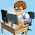# Arithmetic, Smallest And Largest Program In Java

The program below is the answer to Deitel's Java How to Program (9th Edition) Chapter 2 Exercise 2.17.

Question: Write an application that inputs three integers from the user and displays the sum, average, product, smallest and largest of the numbers. Use the techniques shown in Fig. 2.15. [Note: The calculation of the average in this exercise should result in an integer representation of the average. So, if the sum of the values is 7, the average should be 2, not 2.3333….]

```/**
*
* @Author: Aghatise Osazuwa
* Website: www.cscprogrammingtutorials.com
*
* Exercise 2.17 - Arithmetic, Smallest And Largest
* This Program Calculates And Displays The Sum, Average, Product, Smallest And Largest of
* Three Integer Numbers.
*
*/

import java.util.Scanner;

public class Ex02_17 {
public static void main (String [] args) {

Scanner value = new Scanner (System.in);

int num1;
int num2;
int num3;
int sum;
int average;
int product;
int smallest;
int largest;

System.out.print ("Enter Your First Number: ");
num1 = value.nextInt ();
System.out.print ("Enter Your Second Number: ");
num2 = value.nextInt ();
System.out.print ("Enter Your Third Number: ");
num3 = value.nextInt ();

sum = num1 + num2 + num3;
average = (num1 + num2 + num3)/3;
product = num1 * num2 * num3;

smallest = num1; // assume smallest is the first number
if (num2 < smallest)
smallest = num2;
if (num3 < smallest)
smallest = num3;

largest = num1; // assume largest is the first number
if (num2 > largest)
largest = num2;
if (num3 > largest)
largest = num3;

System.out.printf ("\nSum = %d\nAverage = %d\nProduct = %d\nSmallest = %d\n"
+ "Largest = %d\n", sum, average, product, smallest, largest);
}
}
```

1.Thanks for the answers, but you forgot to set int largest; in your answer

1.Thanks for pointing that out. I've corrected it.

DISCLAIMER: Opinions expressed in comments are those of the comment writers alone and does not reflect or represent the views of the post author. We reserve the right to delete any post deemed inappropriate or offensive and/or spammy. Please do not use abusive words/hate speech.

I Appreciate your valuable Feedback. So, Please DO NOT SPAM - Spam comments will be deleted immediately.

Don't use brand name in name field and you're not allowed to use links in comments unless it's necessary. Such comments will be removed immediately. To get notified of replies or follow-up comments, click the box next to notify me.

Thanks.